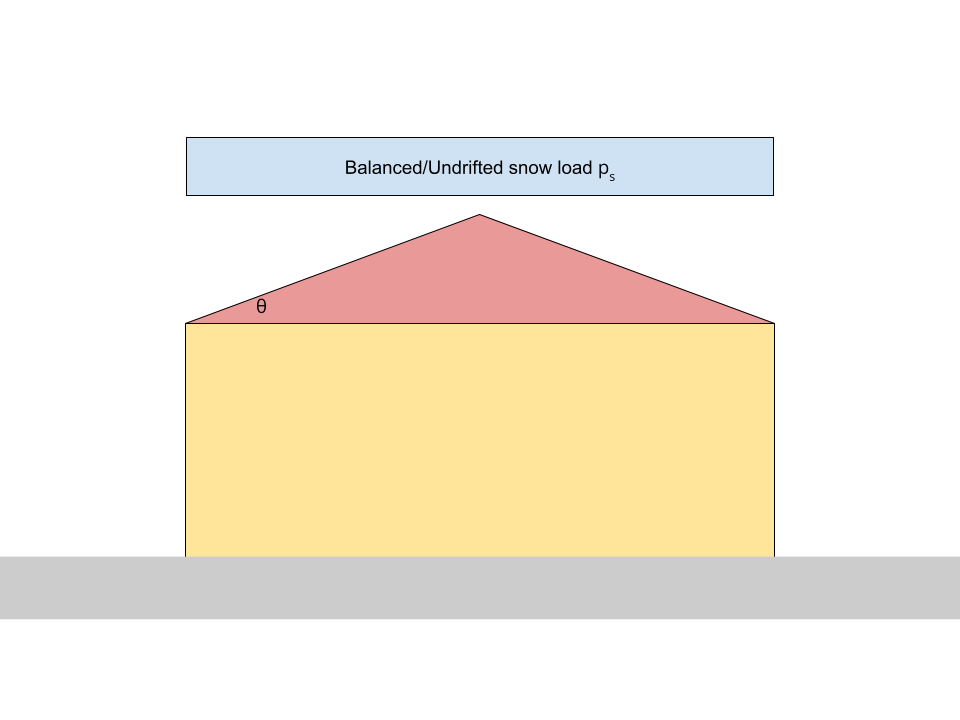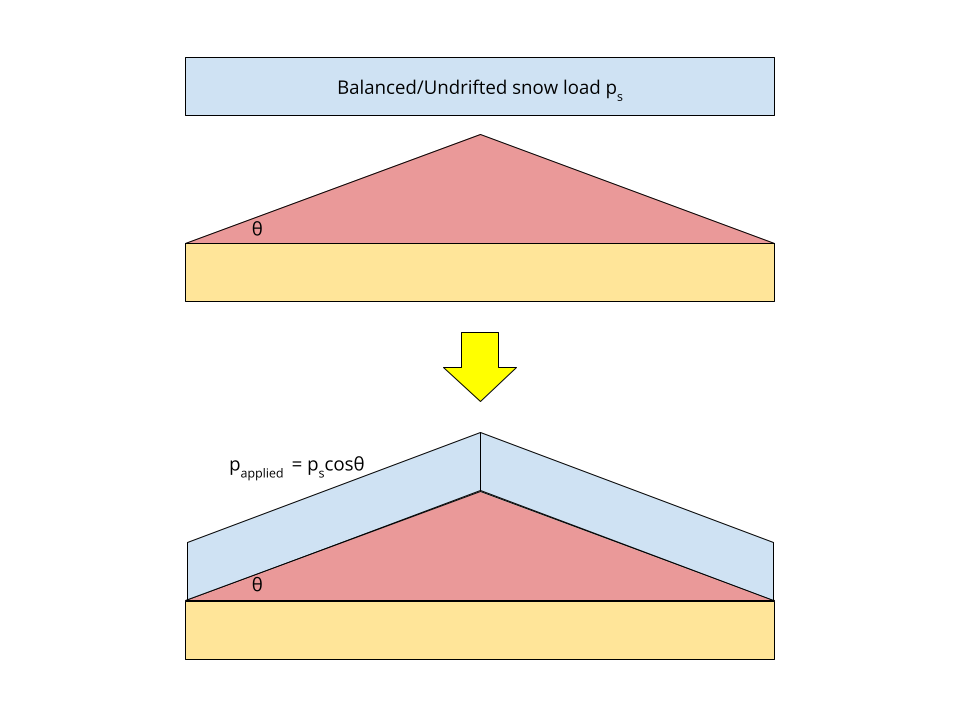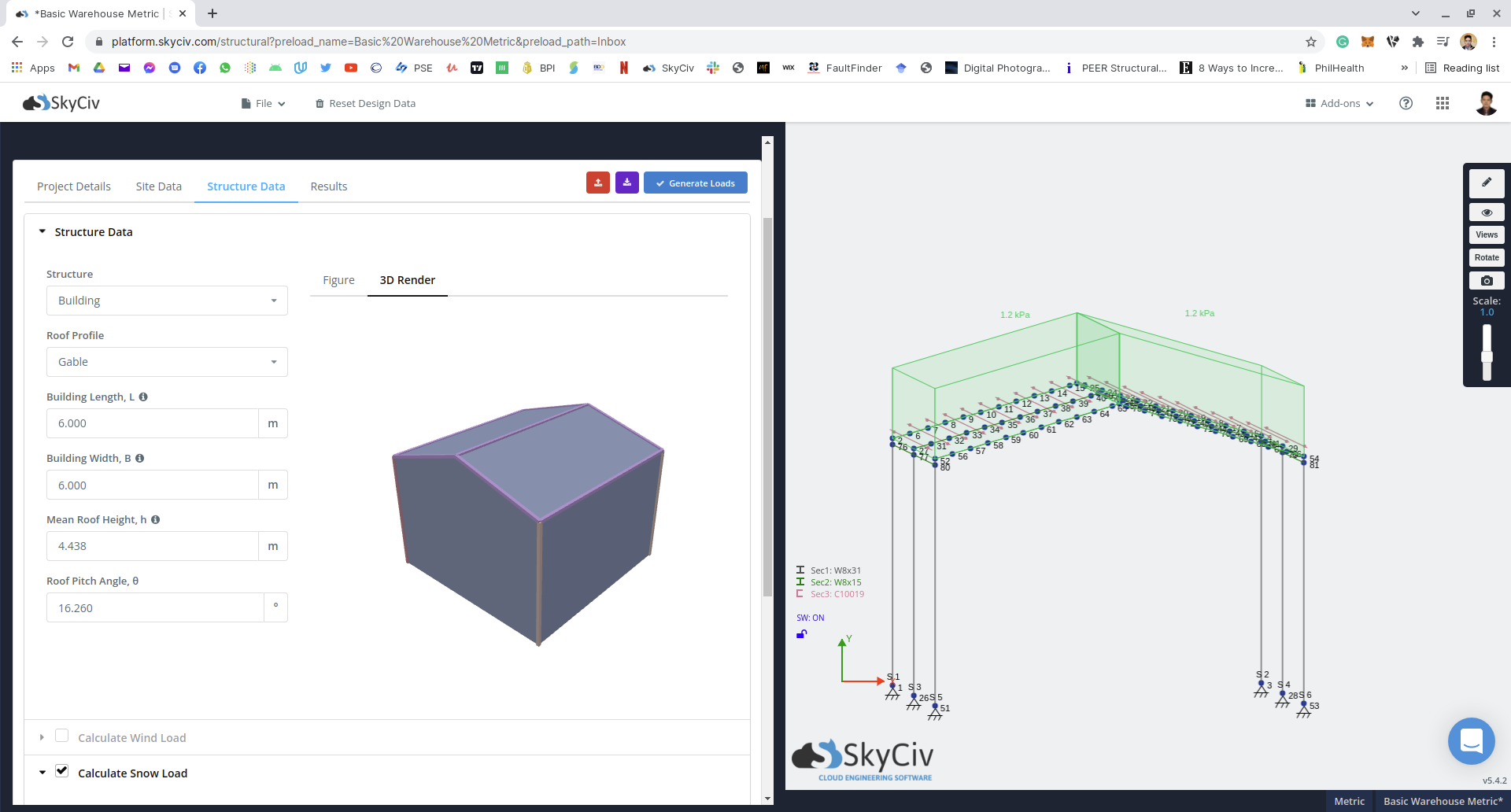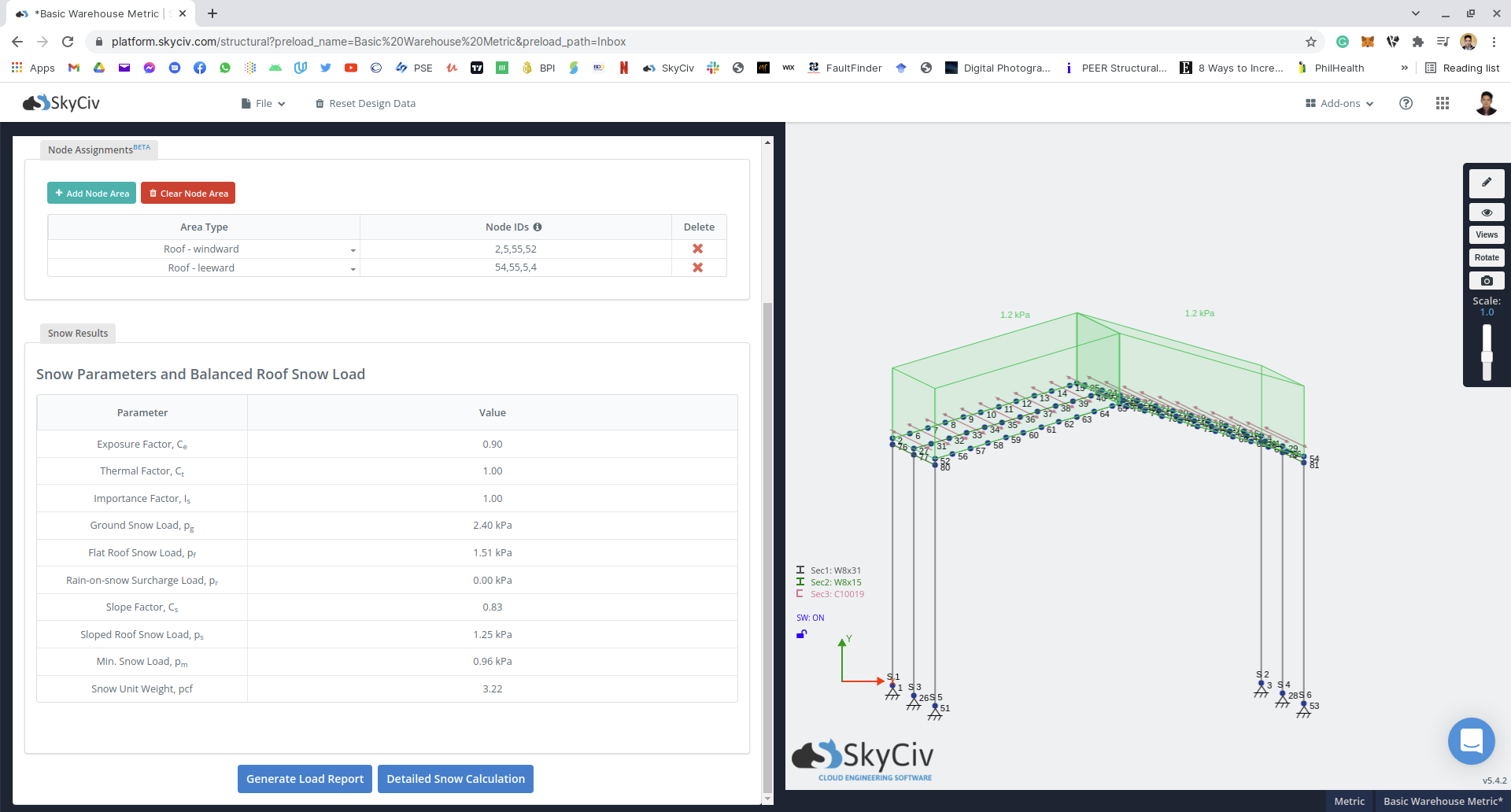Documentación SkyCiv

Su guía para el software SkyCiv - tutoriales, guías prácticas y artículos técnicos

1. Inicio
3. Cargas de nieve
4. Carga de nieve integrada en SkyCiv S3D

# Carga de nieve integrada en SkyCiv S3D

In calculating snow loads, the balanced or undrifted snow load on roof is always assumed to be applied on the horizontal projection of the roof area the same way live loads are applied.In order to apply the snow load on the roof, we need to convert pressure $${Precios}_ _{s}$$ by multiplying it by the value of cosθ.## Using SkyCiv Load Generator in S3D

por Usuarios de cuentas profesionales, you can access the Load Generator thru the area load sections in S3D. From the module, generating the balanced/undrifted snow load $${Precios}_ _{s}$$ is automatically applied as long as the roof windward and leeward nodes are defined the the Node Assignments mesa.The rule to define the roof windward and leeward nodes is shown below:Por lo tanto, the corresponding snow load applied is shown below:From this model, the balanced/undrifted snow load $${Precios}_ _{s} = 1.25 kPa$$ is converted by multiplying the cosine value of the Roof Pitch Angle θ igual a 0.9603. Por lo tanto, the corresponding roof snow load is equal to $$p = 1.20kPa$$. Using open area load, it is then applied to the roof surface of the model. For the unbalanced/drifted case, manual input is necessary. This feature is only available to ASCE 7-10, ASCE 7-16, NBCC 2015, EN 1991, y AS / NZS 1170.Patrick Aylsworth García
Ingeniero estructural, Desarrollo de Producto
Maestría en Ingeniería Civil